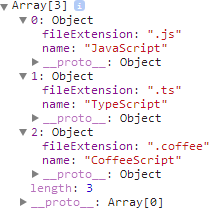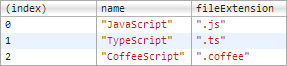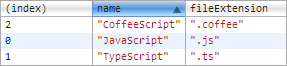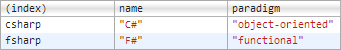### 封装

``` var Cat = { name : '', color : '' }```

``` var cat1 = {}； cat1.name = '大毛'; cat1.color = '黄色'; var cat2 = {}; cat2.name = '二毛'; cat2.color = '黑色';```

``` function Cat(name,color){ return { name : name, color : color } }```

``` var cat1 = Cat('大毛','黄色'); var cat2 = Cat('二毛','黑色');```

``` function Cat(name,color){ this.name = name; this.color = color; }```

``` var cat1 = new Cat('大毛','黄色'); var cat2 = new Cat('二毛','黑色');```

``` console.log(cat1 instanceof Cat); //true console.log(cat2 instanceof Cat); //true```

``` function Cat(name,color){ this.name = name; this.color = color; this.type = '猫科动物'; this.eat = function(){console.log('吃老鼠');} }```

``` var cat = Cat('大毛','黄色'); console.log(cat.type); // 猫科动物 cat.eat(); // 吃老鼠。```

prototype 模式

``` function Cat(name,color){ this.name = name; this.color = color; } Cat.prototype.type = '猫科动物'; cat.prototype.eat = function(){console.log('吃老鼠');}```

``` var cat1 = new Cat('大毛','黄色'); var cat2 = new Cat('二毛','黑色');```

``` console.log(cat1.eat === cat2.eat); //true```

prototype 模式的验证方法

``` isPrototypeOf()```

``` console.log(Cat.prototype.isPrototypeOf(cat1)); //true```

hasOwnProperty()

``` console.log(cat1.hasOwnProperty('name')); true console.log(cat1.hasOwnProperty('type')); false```

in 运算符

``` console.log("name" in cat1); // true console.log('type' in cat1); // true console.log('typo' in cat1); // false```

in 运算符还可以用来遍历对象的属性。

``` for(var key in cat1){ console.log('cat1[' + key ' ]=' + cat1[key]); }```

#### 兼容多种模块规范的 javascript 模块化写法

``` ;(function(name,definition){ var hasDefine = typeof define === 'function', // 检测上下文环境是否为 AMD 或者 CMD hasExports = typeof module !== 'undefined' && module.exports; // 检查上下文是否为 node if(hasDefine){ define(definition); // AMD 或者 CMD 环境 }else if(hasExports){ module.exports = definition(); // 定义为普通 node 模块 }else{ this[name] = definition(); // 将模块的执行结果挂载在 window 变量中，在浏览器中 this 指向 window 变量。 } })('hello',function(){ // 此处 hello 只是作为模块化的示例名称 var hello = function(){}; return hello; })```

#### JavaScript 中的 sort 方法

sort 作为数组的方法，被定义在 Array.prototype.sort，也即在 JavaScript 中所有数组都可以使用 sort 方法进行排序。虽然一般情况下，sort 甚至可以不用传递排序函数一样可以正常工作，但很多情况下，我们还是必须明确的定义排序函数，才能更好的按我们的需求来工作，这也是一般情况下建议的方式，因为不知道不同的 js 引擎在不传递排序函数的时候，会按照什么方案进行排序。

``` var colors = ['red', 'green', 'blue', 'yellow', 'white']; console.log(colors.sort()); // ["blue", "green", "red", "white", "yellow"] console.log(colors); // ["blue", "green", "red", "white", "yellow"]```

``` var colors = ['red', 'green', 'blue', 'Yellow', 'White']; console.log(colors.sort()); // ["White", "Yellow", "blue", "green", "red"]```

``` var money = [12, 3, 7.4, 200]; console.log(money.sort()); // [12, 200, 3, 7.4]```

``` var money = [12, 3, 7.4, 200]; var compare = function(a, b) {return a - b;}; console.log(money.sort(compare)); // [3, 7.4, 12, 200] //console.log(money.sort(function(a, b) {return a - b;})); // [3, 7.4, 12, 200]```

``` var people = [ { name: 'Alice', id: 1234 }, { name: 'Bob', id: 567 } ]; var compare = function(a, b) {return a.id - b.id;} console.log(people.sort(compare)); // Bob is before Alice now```

``` var everything = [4, 'Red', '\$200', 'white', 7.4, 12, true, 0.3, false]; var compare = function(a, b) {return a - b;}; console.log(everything.sort(compare));```

#### 使用 JavaScript 的 console.table() 进行 debug

``` var languages = [ { name: "JavaScript", fileExtension: ".js" }, { name: "TypeScript", fileExtension: ".ts" }, { name: "CoffeeScript", fileExtension: ".coffee" } ]; console.log(languages);`````` var languages = { csharp: { name: "C#", paradigm: "object-oriented" }, fsharp: { name: "F#", paradigm: "functional" } }; console.table(languages);`````` console.table(languages, ["name", "paradigm"]); //多个 key 组成的数组```

``` console.table(languages, "name”); //单个 key```

#### Javascript 中的 Bind ，Call 以及 Apply

``` var bind = Function.prototype.call.bind(Function.prototype.bind);```

``` var context = {foo : “bar”};```

``` function returnFoo(){ return this.foo; }```

（具体解释为，此时， this 指代全局的 window，而 window.foo 没有定义，所以返回 undefined）

``` returnFoo(); // undefined```

``` var bound = returnFoo.bind(context);```

（即，在 context 这个上下文中，是有定义 foo 变量的）

``` bound(); // bar```

``` returnFoo.call(content); // bar returnFoo.apply(content); // bar```

``` content.returnFoo = returnFoo; content.returnFoo(); // bar```

Array.prototype 有一个特别好用的方法，叫做 slice。

``` [1,2,3].slice(0,1); // ```

``` var slice = Array.prototype.slice;```

slice 现在未被绑定到任何对象，所以它和 Array.prototype.slice 比起来，对提供给它的上下文，或者 this，是不能正常的工作。

``` slice(0,1); //typeError slice([1,2,3],0,1); //TypeError```

``` slice.call([1,2,3],0,1); //  slice.apply([1,2,3],[0,1]); //```

``` slice = Function.prototype.call.bind(Array.prototype.slice); slice([1,2,3],0,1); //```

``` var bind = Function.prototype.call.bind(Function.prototype.bind); var content = {foo : ”bar”}; function returnFoo(){ return this.foo; } var amazing = bind(returnFoo, content); amazing(); //bar```

# 回调与作用域

``` a(b);```

``` b(parameters);```

``` var app = {}; app.number = 1; app.add = function (n) { n = n + this.number; };```

makeArray 函数做了类似下面的事：

``` var makeArray = function (callback) { // ... if (typeof callback === "function") { callback(i); } // ... };```

``` makeArray(app.add);```

``` makeArray(app.add, app);```

``` var makeArray = function (callback, callback_obj) { //... if (typeof callback === "function") { callback.call(callback_obj, i); } // ... };```

``` makeArray(app.add, app);```

``` makeArray("add", app);```

``` var makeArray = function (callback, callback_obj) { if (typeof callback === "string") { callback = callback_obj[callback]; } //... if (typeof callback === "function") { callback.call(callback_obj, i); } // ... };```

# 匿名的事件监听器

``` document.addEventListener("click", console.log, false);```

# 定时

``` var thePlotThickens = function () { console.log('500ms later...'); }; setTimeout(thePlotThickens, 500);```

``` setTimeout(function(){thePlotThickens()}, 500);```